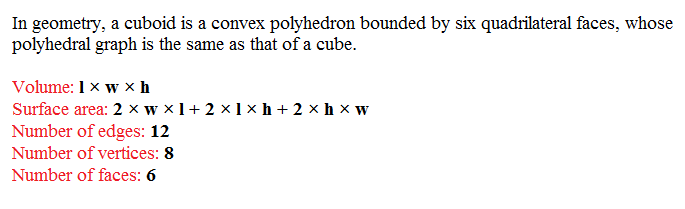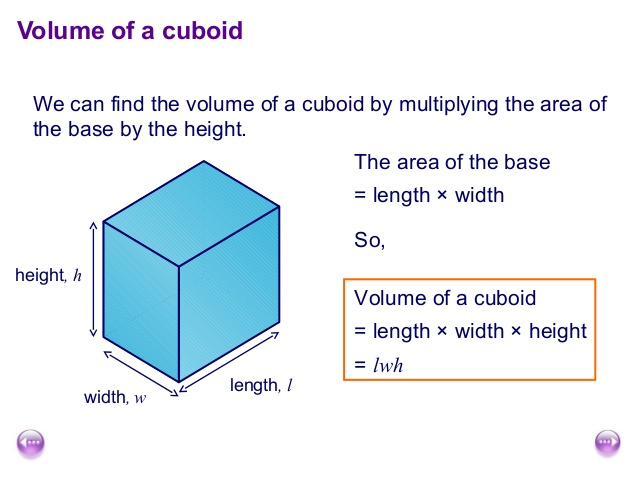# Volume Of Cuboid : Java Program In 3 Simple Ways

Java program to calculate the volume of a cuboid. We can find the value of cuboid in so different ways. We do written the code in 3 different ways check out the table of contents so that you will get an idea. If you have any doubts related to the following java program to find the volume of cuboid, then do comment our dedicated team will help you out.

Before we explain the code, a couple of lines about cuboid and math formula to find the volume.

What is Cuboid?

A: Convex Polyhedron which was bounded by 6 Quadrilateral Faces.#Cuboid Def

What is the Formula To Find the Volume of Cuboid?

A: Finding the volume is quite easy. Simple formula Is L X B X H. Most of the code will be base on the following Formula. Do check out 🙂Sample Program # 1:

Here Goes the Sample Program if you know the basics then skip the explanation part and move on to the second sample program to find the volume of a cuboid. # Do check out the Online execution and compiler tool just after the code.

Output :

Here Goes the online implementation tool for the above sample program # 1. Enter the values by line by line. If you encounter any problems while executing the program, do let us know.

Here goes the another sample program.

Sample Program #2: To calculate a volume of cuboid ( Using command line arguments )

What are command line arguments?